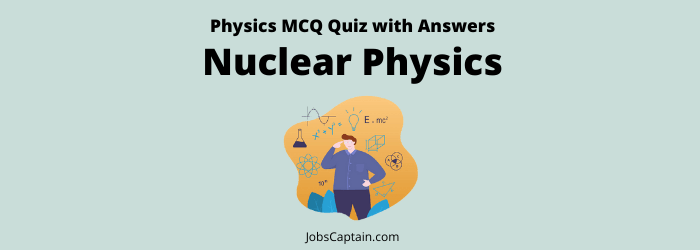# Nuclear Physics MCQ Quiz 1Quiz Set 1 2 3

Question 1. One of these particles is claimed to have invented which rebut the Einstein’s theory of relativity.

(A) Light emitting diode

(B) Liquid crystal

(C) Neutrino

(D) Micro-wave Photon

(D) Micro-wave Photon

Question 2. Cyclotrons are used to accelerate.

(A) Ions

(B) Atoms

(C) Protons

(D) Neutrons

(C) Protons

Question 3. Which one of the following pairs is not correctly matched?

(A) Synthesis of transuranic elements – Enrico Fermi

(B) Theory of energy production in the Sun and stars – H.A. Bethe

(C) Discovery of Positron – C.D. Anderson and U.F. Hess

(D) Discovery of Meson – Hideki Yukawa

(A) Synthesis of transuranic elements – Enrico Fermi

Question 4. Which one of the following is not correct?

(A) Telephone was invented by Graham Bell

(B) ‘Dry ice’ is nothing but solid carbon dioxide

(C) The breaking apart of the nucleus of an atom is called fusion

(D) Theory of evolution was propounded by Charles Darwin

(C) The breaking apart of the nucleus of an atom is called fusion

Question 5. Which one of the following can be used to confirm whether drinking water contains a gamma emitting isotope or not?

(A) Spectrophotometer

(B) Scintillation counter

(D) Microscope

(B) Scintillation counter

Question 6. Among the following radiations, which has the highest energy?

(A) Infra-red

(B) Ultra-violet

(C) X-ray

(D) Visible

(C) X-ray

Question 7. The energy of Sun is released due to_______.

(A) Reduction reactions

(B) Oxidation reactions

(C) Nuclear fusion

(D) Nuclear fission

(C) Nuclear fusion

Question 8. The stars receive their energy from which of the following?

(A) Gravitational pull

(B) Chemical reaction

(C) Nuclear fission

(D) Nuclear fusion

(D) Nuclear fusion

Question 9. Which one of the following is the source of renewable energy in stars?

(A) Excess of oxygen which is helpful in burning and generate energy

(C) Helium changes into hydrogen

(D) Hydrogen changes into helium

(D) Hydrogen changes into helium

Question 10. Source of energy from the Sun is_______.

(A) Cherenkov effect

(B) Photoelectric effect

(C) Nuclear fusion

(D) Nuclear fission

(C) Nuclear fusion

Question 11. What is the source of electrical energy in an artificial satellite?

(A) Thermopile

(B) Dynamo

(C) Mini nuclear reactor

(D) Solar cells

(D) Solar cells

Question 12. Which one of the following element is used in solar cells?

(B) Cerium

(C)  Astatine

(D) Silicon

(D) Silicon

Question 13. Which one of the following cannot be used as a nuclear fuel?

(A) Plutonium

(B) Calcium

(C) Thorium

(D) Uranium

(B) Calcium

Question 14. Which of the following is not a fuel element?

(A) Helium

(C) Thorium

(D) Uranium

(A) Helium

Question 15. Identify the mineral not associated with atomic power.

(A)  Chromium

(B) Beryllium

(C) Thorium

(D) Monazite

(A)  Chromium

Question 16. Which of the following element is not included as a nuclear fuel?

(A) Uranium

(B) Plutonium

(C) Thorium

Question 17. India is an important member of the ‘International Thermonuclear Experimental Reactor’. If this experiment succeeds, what is the immediate advantage for India?

(A) It can build fusion reactors for power generation

(B) It can drastically improve the efficiency of its fission reactors in power generation

(C) It can attain a global role in satellite navigation

(D) It can use thorium in place of uranium for power generation

(A) It can build fusion reactors for power generation

Question 18. Radioactive element which has been found to have large reserves in India is________.

(A) Uranium

(B) Thorium

(D) Plutonium

(B) Thorium

Question 19. The important nuclear fuel available in India in abundance is_________.

(A) Plutonium

(B)  Iridium

(C) Thorium

(D) Uranium

(C) Thorium

Question 20. In the modern day’s nuclear medicine plays a vital role in Medical Science, it is exactly______.

(A) They are chlorofluorocarbons

(C) They are infusion of herbs

(D) They are drugs

Question 21. The difference between nuclear reactor and atom bomb is that_______.

(A) Atom bomb is based on nuclear fusion whereas in nuclear reactor, nuclear fission occurs

(B) The chain reaction in nuclear reactor is not controlled

(C) The chain reaction in nuclear reactor is controlled

(D) There is no chain reaction in nuclear reactor whereas this happens in atom bomb

(C) The chain reaction in nuclear reactor is controlled

Question 22. What is Nuclear Reactor?

(A) Molecular furnace

(B) The emitter of U238

(C) Heavy water pond

(D) Place where atomic bomb is built

(A) Molecular furnace

Question 23. Which statement is not associated with nuclear fission?

(A) Enormous energy is released in an atom bomb

(B) Source of energy in nuclear reactor that produces electricity

(C) Energy generation in stars

(D) Neutron-induced nuclear reaction

(C) Energy generation in stars

Question 24. Photovoltaic cell is related to________.

(A) Wind Energy

(B) Solar Energy

(C) Geo Thermal Energy

(D) Nuclear Energy

(B) Solar Energy

Question 25. Photovoltaic cells are_________.

(A) Molar cells

(B) Sulphur cells

(C) Thermal cells

(D) Solar cells